• +91 9971497814
• info@interviewmaterial.com

# Chapter 6 Linear Inequalities Ex-6.3 Interview Questions Answers

### Related Subjects

Question 1 :

Solve the following system ofinequalities graphically: x ≥ 3, y ≥ 2

x ≥ 3 … (1)
y ≥ 2 … (2)
The graph of the lines, x = 3 and y = 2, are drawn in the figure below.
Inequality (1) represents the region on the right hand side of the line, x = 3 (including the line x = 3), and inequality (2) represents the region above the line, y = 2 (including the line y = 2).
Hence, the solution of the given system of linear inequalities is represented by the common shaded region including the points on the respective lines as follows.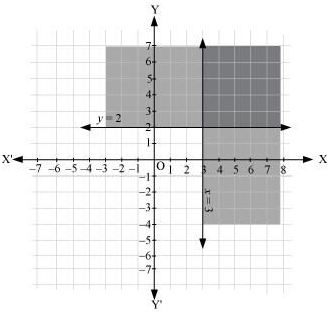Question 2 : Solve the following system of inequalities graphically: 3x + 2y ≤ 12, x ≥ 1, y ≥ 2

3x + 2y ≤ 12 … (1)
x ≥ 1 … (2)
y ≥ 2 … (3)
The graphs of the lines, 3x + 2y = 12, x = 1, and y = 2, are drawn in the figure below.
Inequality (1) represents the region below the line, 3x + 2y = 12 (including the line 3x + 2y = 12). Inequality (2) represents the region on the right side of the line, x = 1 (including the line x = 1). Inequality (3) represents the region above the line, y = 2 (including the line y = 2).
Hence, the solution of the given system of linear inequalities is represented by the common shaded region including the points on the respective lines as follows.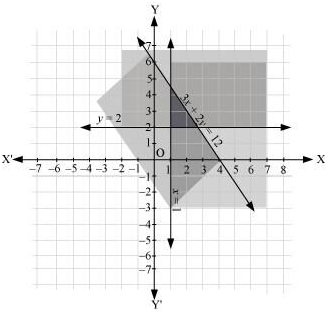Question 3 : Solve the following system of inequalities graphically: 2x + y≥ 6, 3x + 4y ≤ 12

2x + y≥ 6 … (1)
3x + 4y ≤ 12 … (2)
The graph of the lines, 2x + y= 6 and 3x + 4y = 12, are drawn in the figure below.
Inequality (1) represents the region above the line, 2x + y= 6 (including the line 2x + y= 6), and inequality (2) represents the region below the line, 3x + 4y =12 (including the line 3x + 4y =12).
Hence, the solution of the given system of linear inequalities is represented by the common shaded region including the points on the respective lines as follows.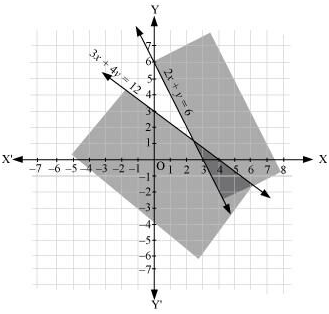Question 4 : Solve the following system of inequalities graphically: x + y≥ 4, 2x – y > 0

x + y≥ 4 … (1)
2x – y > 0 … (2)
The graph of the lines, x + y = 4 and 2x – y = 0, are drawn in the figure below.
Inequality (1) represents the region above the line, x + y = 4 (including the line x + y = 4).
It is observed that (1, 0) satisfies the inequality, 2x – y > 0. [2(1) – 0 = 2 > 0]
Therefore, inequality (2) represents the half plane corresponding to the line, 2x – y = 0, containing the point (1, 0) [excluding the line 2x – y > 0].
Hence, the solution of the given system of linear inequalities is represented by the common shaded region including the points on line x + y = 4 and excluding the points on line 2x – y = 0 as follows.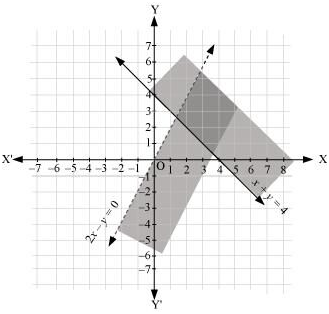Question 5 : Solve the following system of inequalities graphically: 2x – y > 1, x – 2y < –1

2x – y > 1 … (1)
x – 2y < –1 … (2)
The graph of the lines, 2x – y = 1 and x – 2y = –1, are drawn in the figure below.
Inequality (1) represents the region below the line, 2x – y = 1 (excluding the line 2x – y = 1), and inequality (2) represents the region above the line, x – 2y = –1 (excluding the line x – 2y = –1).
Hence, the solution of the given system of linear inequalities is represented by the common shaded region excluding the points on the respective lines as follows.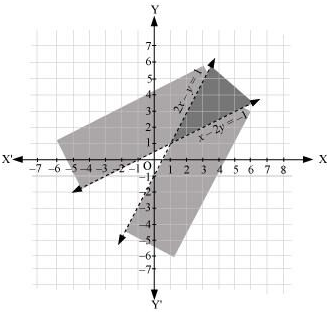Question 6 : Solve the following system of inequalities graphically: x + y ≤ 6, x + y ≥ 4

x + y ≤ 6 … (1)
x + y ≥ 4 … (2)
The graph of the lines, x + y = 6 and x + y = 4, are drawn in the figure below.
Inequality (1) represents the region below the line, x + y = 6 (including the line x + y = 6), and inequality (2) represents the region above the line, x + y = 4 (including the line x + y = 4).
Hence, the solution of the given system of linear inequalities is represented by the common shaded region including the points on the respective lines as follows.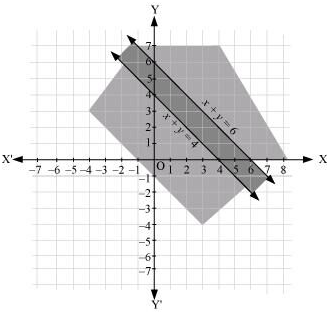Question 7 : Solve the following system of inequalities graphically: 2x + y≥ 8, x + 2y ≥ 10

2x + y= 8 … (1)
x + 2y = 10 … (2)
The graph of the lines, 2x + y= 8 and x + 2y = 10, are drawn in the figure below.
Inequality (1) represents the region above the line, 2x + y = 8, and inequality (2) represents the region above the line, x + 2y = 10.
Hence, the solution of the given system of linear inequalities is represented by the common shaded region including the points on the respective lines as follows.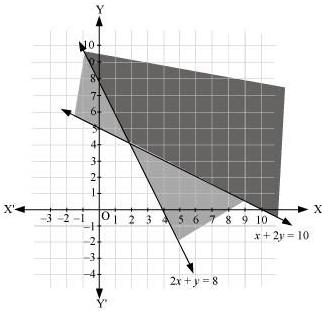Question 8 : Solve the following system of inequalities graphically: x + y ≤ 9, y > x, x ≥ 0

x + y ≤ 9      ... (1)
y > x             ... (2)
x ≥ 0             ... (3)
The graph of the lines, x + y= 9 and y = x, are drawn in the figure below.
Inequality (1) represents the region below the line, x + y = 9 (including the line x + y = 9).
It is observed that (0, 1) satisfies the inequality, y > x. [1 > 0]
Therefore, inequality (2) represents the half plane corresponding to the line, y = x, containing the point (0, 1) [excluding the line y = x].
Inequality (3) represents the region on the right hand side of the line, x = 0 or y-axis (including y-axis).
Hence, the solution of the given system of linear inequalities is represented by the common shaded region including the points on the lines, x + y = 9 and x = 0, and excluding the points on line y = x as follows.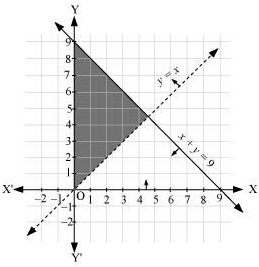Question 9 : Solve the following system of inequalities graphically: 5x + 4y ≤ 20, x ≥ 1, y ≥ 2

5x + 4y ≤ 20 … (1)
x ≥ 1 … (2)
y ≥ 2 … (3)
The graph of the lines, 5x + 4y = 20, x = 1, and y = 2, are drawn in the figure below.
Inequality (1) represents the region below the line, 5x + 4y = 20 (including the line 5x + 4y = 20). Inequality (2) represents the region on the right hand side of the line, x = 1 (including the line x = 1). Inequality (3) represents the region above the line, y = 2 (including the line y = 2).
Hence, the solution of the given system of linear inequalities is represented by the common shaded region including the points on the respective lines as follows.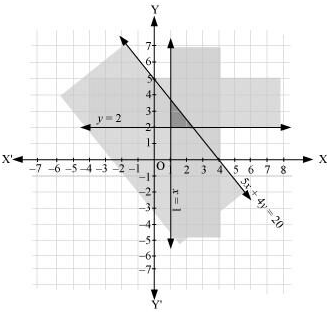Question 10 : Solve the following system of inequalities graphically: 3x + 4y ≤ 60, x + 3y ≤ 30, x ≥ 0, y ≥ 0